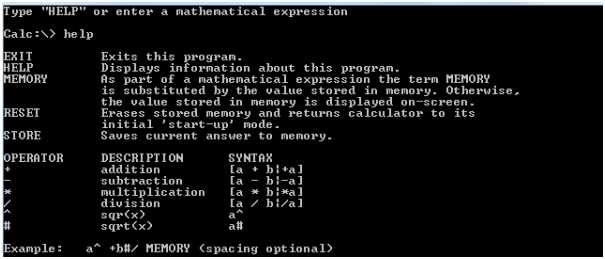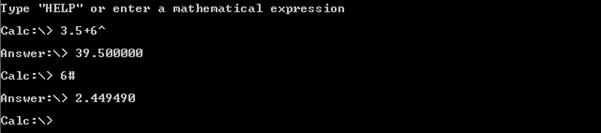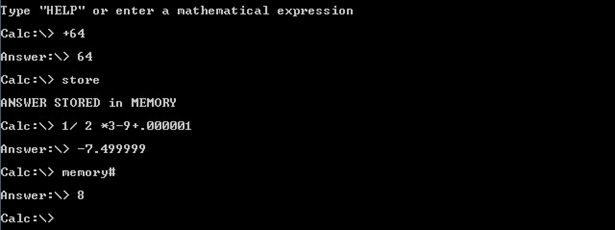HIT365 C Programming Assignment 2 Solved

25.00 \$ 12.50 \$

Category:
Click Category Button to View Your Next Assignment | Homework

Description

For this assignment, you are required to develop a non-scientific calculator using C. Your calculator should only solve summation, subtraction, division, product, square root and square expressions. Utilizing the math.h header for your program, your calculator should start by prompting the user to enter a two-operand mathematical equation (for example 3.74 + 22 or 35.32 – 6.2) just like how you would using a non-scientific calculator. Your program should then display the correct answer and the user will have the choice to either continue using the calculator with the current answer to perform further calculations or end the program. Your program should also have a help function to display all the functions of the calculator. A sample output of the program when the user enters “help” is shown below:Your calculator must have the following additional functionalities and features:

1. Your calculator should display values accurate up to 6 decimal places.
2. For square and square root operations, you may define any symbol to represent the operations, but the user should be aware of the symbols you used.
3. Have a memory function to store the current answer and can be used later for further calculations and reference.
4. Have a refresh function to erase the stored memory and return the calculator to its initial

‘start-up’ mode.

Below are some examples of the program output: